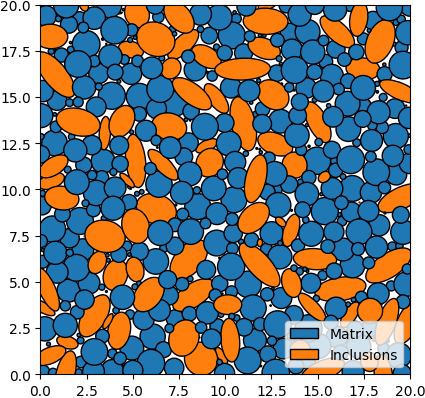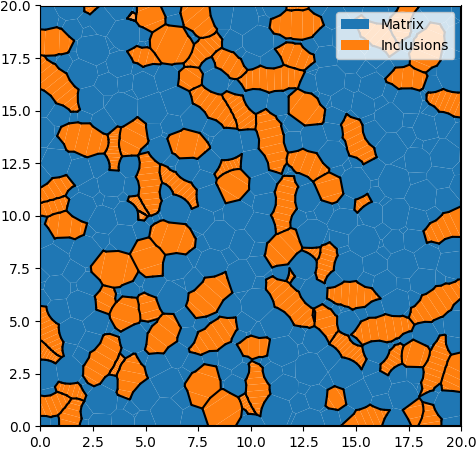# 3. Size & Shape¶

## 3.1. XML Input File¶

The basename for this file is `intro_3_size_shape.xml`. The file can be run using this command:

```microstructpy --demo=intro_3_size_shape.xml
```

The full text of the file is:

```<?xml version="1.0" encoding="UTF-8"?>
<input>
<material>
<name> Matrix </name>
<material_type> matrix </material_type>
<fraction> 2 </fraction>

<shape> circle </shape>
<size>
<dist_type> uniform </dist_type>
<loc> 0 </loc>
<scale> 1.5 </scale>
</size>
</material>

<material>
<name> Inclusions </name>
<fraction> 1 </fraction>
<shape> ellipse </shape>
<size>
<dist_type> triang </dist_type>
<loc> 0 </loc>
<scale> 2 </scale>
<c> 1 </c>
</size>
<aspect_ratio>
<dist_type> uniform </dist_type>
<loc> 1 </loc>
<scale> 2 </scale>
</aspect_ratio>
<angle> random </angle>
</material>

<domain>
<shape> square </shape>
<side_length> 20 </side_length>
<corner> (0, 0) </corner>
</domain>

<settings>
<directory> intro_3_size_shape </directory>
<verbose> True </verbose>
</settings>
</input>
```

## 3.2. Materials¶

There are two materials, in a 2:1 ratio based on volume. The first is a matrix, which is represented with small circles.

The second material consists of elliptical inclusions with size ranging from 0 to 2 and aspect ratio ranging from 1 to 3. Note that the size is defined as the diameter of a circle with equivalent area. The orientation angle of the inclusions are random, specifically they are uniformly distributed from 0 to 360 degrees.

## 3.3. Domain Geometry¶

These two materials fill a square domain. The bottom-left corner of the rectangle is the origin, which puts the rectangle in the first quadrant. The side length is 20, which is 10x the size of the inclusions to ensure that the microstructure is statistically representative.

## 3.4. Settings¶

Many settings have been left to their defaults, with the exceptions being the verbose mode and output directory.

By default, MicroStructPy does not print status updates to the command line. Switching the verbose mode on will regularly print the status of the code.

The output directory is a filepath for writing text and image files. By default, MicroStructPy outputs texts files containing data on the seeds, polygon mesh, and triangular mesh as well as the corresponding image files, saved in PNG format.

Note

The `<directory>` field can be an absolute or relative filepath. If it is relative, outputs are written relative to the input file, not the current working directory.

## 3.5. Output Files¶

The three plots that this file generates are the seeding, the polygon mesh, and the triangular mesh. These three plots are shown in Fig. 3.1 - Fig. 3.3.Fig. 3.1 Introduction 3 - seed geometries.¶Fig. 3.2 Introduction 3 - polygonal mesh.¶Fig. 3.3 Introduction 3 - triangular mesh.¶